# C 基础

### 1 sizeof

• sizeof实际上是获取了数据在内存中所占用的存储空间，以字节为单位来计数。
• C语言会自动在在双引号""括起来的内容的末尾补上"\0"代表结束，ASCII中的0号位也占用一个字符。
• 是静态运算符，他的结果在编译时刻就决定了。
• 不要在sizeof里做运算，这些运算时不会做的，比如sizeof(a++)，结束后a还是原来的值。

### 2 补码

• 补码的意义就是拿补码和原码可以加出一个溢出的0；11111111  +   00000001  = 100000000
• 对于-a,其补码就是(2的n次幂-a), n是位数。也就是按位取反，逐位加1

### 3 浮点数

• 浮点数在计算时是由专门的硬件部件完成的。
• 浮点数的表达包括：sign, exponent, fraction
• 浮点数里定义了0，正负无穷大。
• 数学上数字是连续的。但是浮点数表达时只能取离散的值，所以表达某个数时，取离它最近的那个离散的值。
• 浮点数不能用==判断是否相等。应该用a-b<10E-8的形式

#### 4 运算符优先级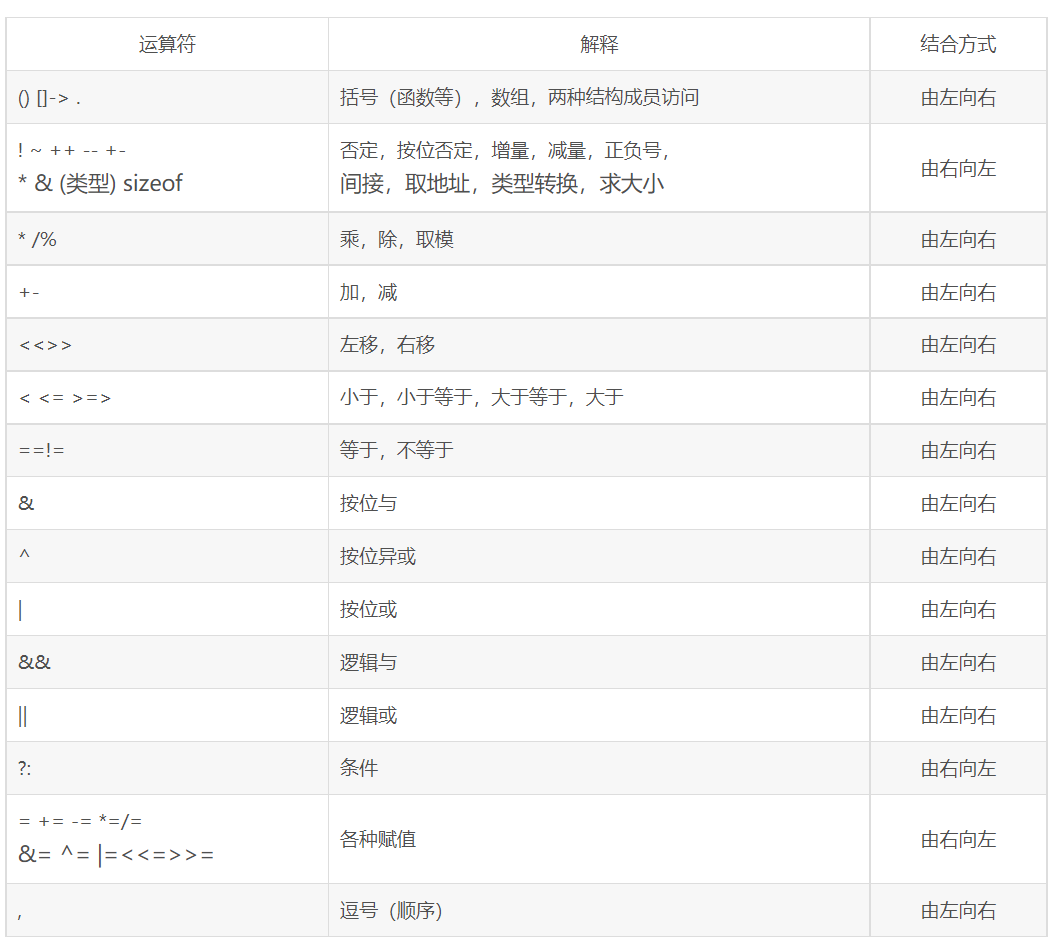1. 累加运算符++，
• a=0; a++，a的值加1，这个表示式的值=a原来的值=0；
• a=0；++a，a的值加1，这个表达式的值=a原来值+1=1；

2. 逗号

• 逗号运算符的优先级是所有运算符中最低的。
• 逗号运算符允许将多个表达式组合成为一个表达式。这个特点使得它适用于在 for 循环头部初始化或递增多个变量；
• 在初始化列表或函数参数列表中的逗号是列表元素的分隔符，它不是逗号运算符。
• 逗号运算符确保操作数被顺序地处理：先计算左边的操作数，再计算右边的操作数。右操作数的类型和值作为整个表达式的结果。x 2.7sqrt2*x )

### 5 函数

• 函数单一出口原则：return只有一个，虽然可以有多个，但是一个函数逻辑更好。
• C编译器是自上而下分析你的代码，所以函数可以放在主函数上面。
• 规范做法是在主函数上做函数原型声明。函数原型就是告诉编译器函数长什么样子，可以不写参数名称，但最好写。函数声明和定义是分开的。
• C语言调用函数时给的值与参数类型可以不匹配，是弱类型语言。
• printf中%d任何小于int的类型会被转换成int，%f,float会被转换成double。但是scanf不会。

#### 6 数组

• sizeof(a)/sizeof(a)用来算数组长度。a数组，a,&a,&a都可以表示数组首位置的地址。数组中单元的地址时线性递增的。
• 调试代码可以放在{}里，变量定义域不会乱。更小的{}块里，若定义的同名变量，优先用自己定义的（强龙打不过地头蛇）
• 二维数组的第二维要确定大小
1. 数组变量是特殊的指针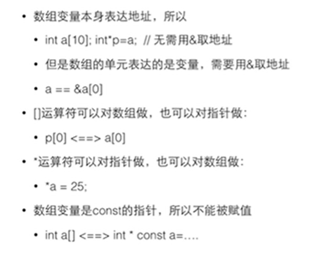2. 指针是const(const在*号后面)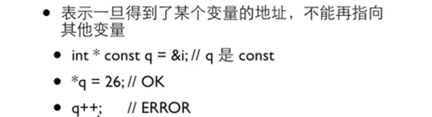3. 所指是const(const在*号之前)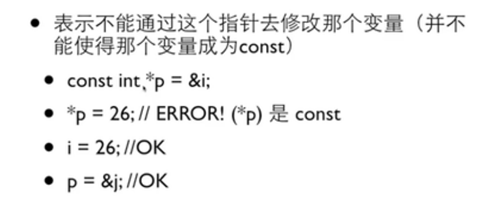• 当要传递的参数的类型比地址大时候，这是常用的手段：既能用比较少的字节数传递值给参数，又能避免函数对外面变量的修改。可用于结构体。

4. const数组

• const int a[] = {1,2}; 表示数组的每个单元都是const int, 所以必须通过初始化赋值。
• 为了保护数组不被函数破坏，可以设置参数为const。int sum(const int a[]), 在函数里a不能修改，在函数外a如果定义时未加const，则可以修改。

#### 7 指针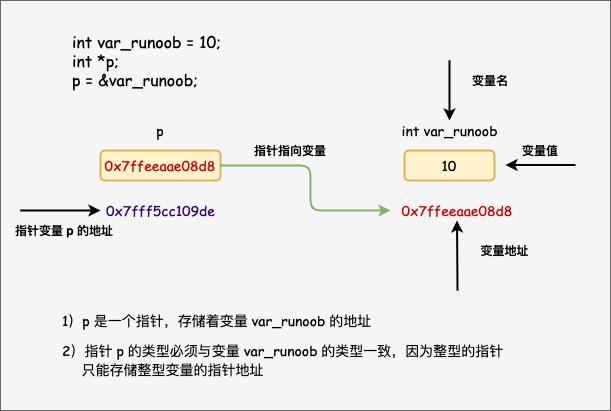1. 指针运算

• 可以给指针加减一个整数；两个指针可以相减；<,<=等比较运算可以对指针做，比较他们在内存中的地址。
• *p++; 故*p++相当于*(p++)，p先与++结合，然后p++整体再与*结合。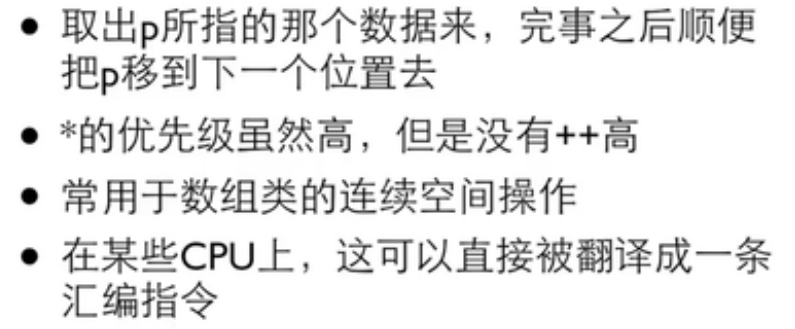2. 指针的类型转换

• void* 表示不知道指向什么东西的指针，
• 转换类型: int* p = &i;  void *q = (void*)p;并没有改变p指的变量的类型，而是通过q看i，把i当作void。

3. 指针用来做什么

• 需要传入较大的数据时用作参数（数组）
• 传入数组后对数组做操作
• 函数返回不止一个结果
• 需要用函数来修改不止一个变量
• 动态申请的内存

4. 0 地址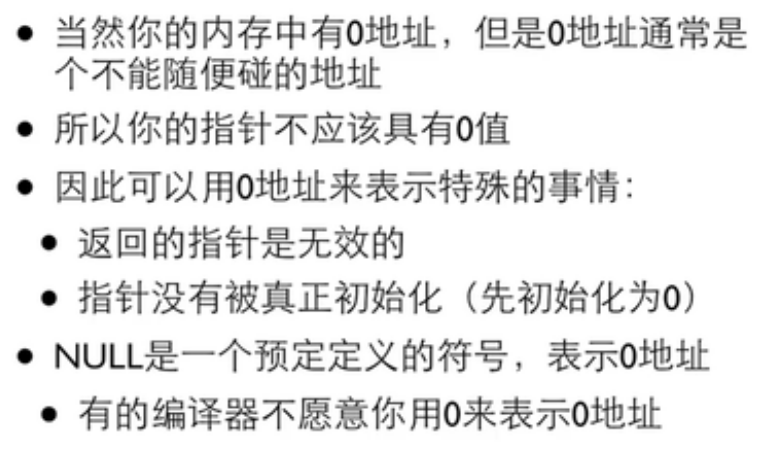5. 作为函数参数的指针

• 指针传入函数，指针本身也是形参，函数内指针是main函数里指针的副本。
void local_data_test(int *plocal_data)
{
plocal_data = (int *)malloc(sizeof(int));
*plocal_data = 20;
printf("Function plocal_data value: %d\n", *plocal_data);
printf("地址%p\n", plocal_data);
printf("地址%p\n", &plocal_data);
}
int main()
{
int *main_data = NULL;
local_data_test(main_data);
printf("地址%p\n", main_data);
printf("地址%p\n", &main_data);
printf("Return data: %d\n", *main_data);
free(main_data);
return 0;
}
•  将函数内部定义的变量用static修饰：由于static修饰的变量，分配在静态内存区（类似于全局变量区），函数返回时，并不会释放内存，因此可以将要返回的变量加static修饰。
• 使用分配在堆上的内存：分配在堆上的内存主要指通过malloc、calloc、realloc等函数动态分配的内存，由于堆上的内存需要手动释放，因此可以在使用完以后再释放，这样指针就不会指向未知。

注意：堆上的内存必须要用完即释放，否则容易造成内存泄漏。

int *local_data_test()
{
int *plocal_data;

plocal_data = (int *)malloc(sizeof(int));
*plocal_data = 20;

printf("Function plocal_data value: %d\n", *plocal_data);

return plocal_data;
}

int main()
{
int *main_data = NULL;

main_data = local_data_test();

printf("Return data: %d\n", *main_data);
free(main_data);
main_data = NULL;

return 0;
}

### 8 动态分配内存

• #include<stdlib,h>   void *malloc(size_t size)，向malloc申请的空间的大小是以字节为单位的；
• 返回的结果是void*，需要类型转换为自己需要的类型（int*)malloc(n*sizeof(int)
• free申请来的还回去，只能还申请来的空间的首地址

### 9 字符串

1. 字符串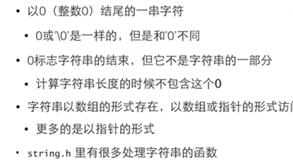•  printf里""两个相邻的“”会合成一个字符串

2. 指针定义的字符串放在代码段，数组定义的放在本地变量的地方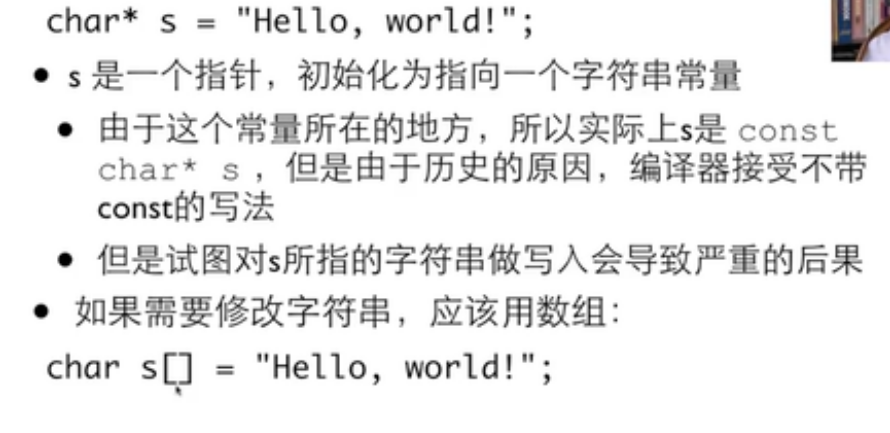• 数组：作为本地变量空间自动被回收；
• 指针：这个字符串不知道在哪里，用来处理参数（参数传入函数），或者动态分配空间。
• 如果要构造字符串用数组；如果要处理字符串用指针（处理指作为参数传入函数）

3. 常见错误：string要分配空间

string没有初始化，本来string里的内容，可能使string指向一个不能被更改的地址。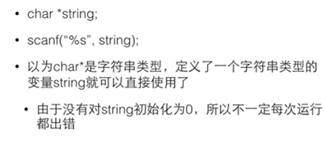4. 空字符串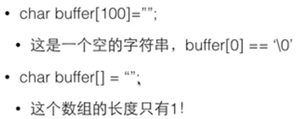5. <string.h>里函数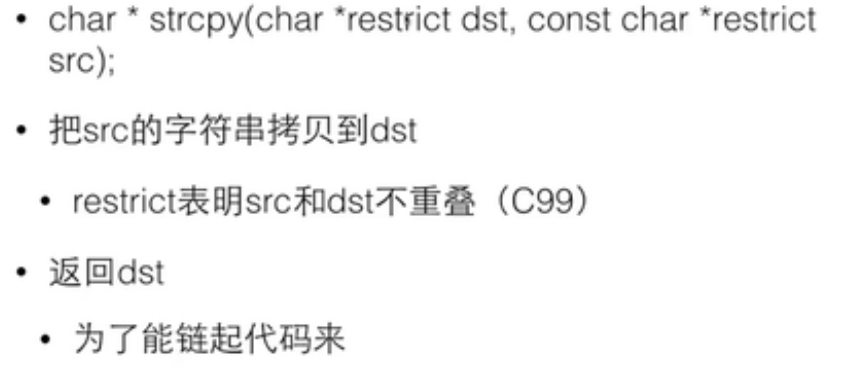• 防止空间不够等问题，指定长度：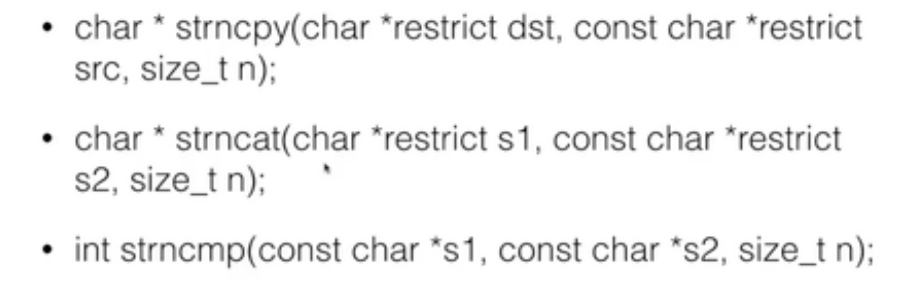char s[] = "hello";
char *p = strchr(s, 'l');//指针指到第一个l位置
p = strchr(p+1, 'l');//从第一个l的下一个位置找下一个l的位置；
char *t = (char *)malloc(strlen(p)+1);//分配空间记得加1
strcpy(t, p);//找到位置之后的数据赋值给t
*p='\0';
strcpy(t,s);//找到位置之前的数据赋值给t;
free(t); 

### 10 枚举

• 枚举量可以作为值来用
• 枚举类型可以跟上enum作为类型，但实际上是以整数来做内部计算和外部输入输出的。
• 声明枚举量可以指定值
• 枚举只是int
enum COLOR {RED, YELLOW,GREEN,NumCOLORS};
int main()
{
int color = -1;
char *colorName[NumCOLORS]={"red","yellow","green"};
char *name=NULL;
printf("输入颜色代码\n");
scanf("%d",&color);
if(color>=0 && color<=NumCOLORS)
{
name=colorName[color];
}
else
{
name="unknow";
}
printf("颜色%s\n",name);
return 0;
} 

### 11 结构体

• 函数内部声明的结构类型只能在函数内部使用；通常在外部声明，就可以被多个函数使用了。
• 声明结构体，声明结构体的变量：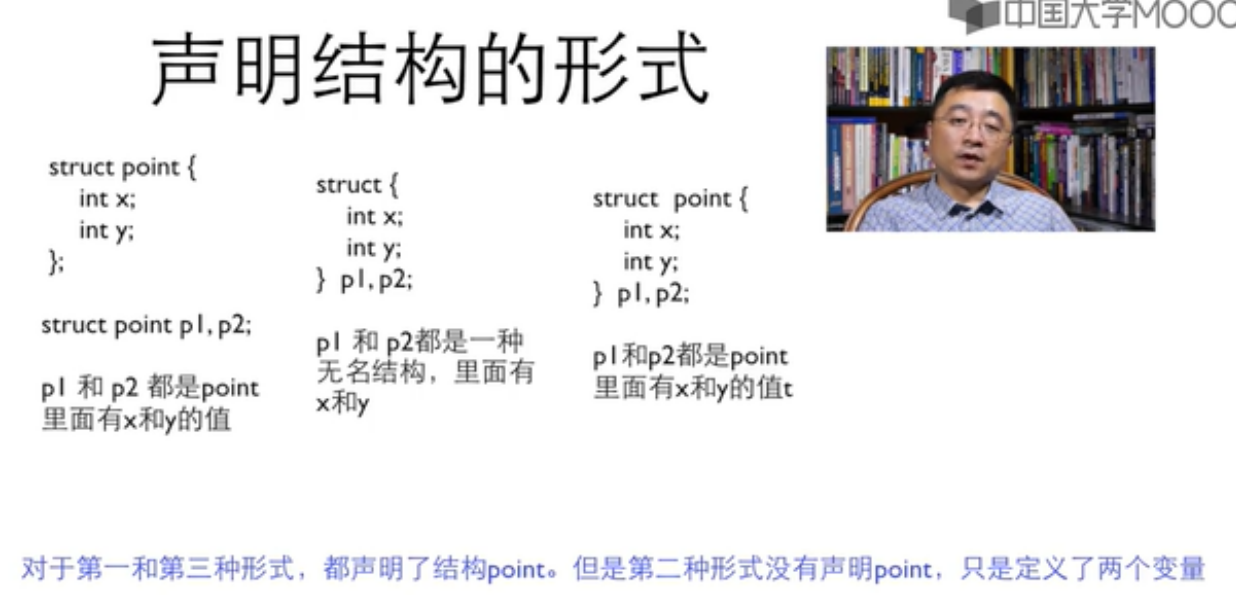struct date{
int month;
int year;
int day;
};
int main()
{
struct date today = {9,2010,3};//初始化
struct date tom = {.month = 9,.year = 2010};//初始化
struct date temp;//声明变量
temp = (struct date){3,2010,8};//赋值
temp = tom;
struct date *pDate = &today;//结构变量的名字并不是结构变量的地址，必须使用&运算符
return 0;
}
• 结构体作为函数参数时，在函数内新建一个结构变量，赋值调用者的结构的值；这种方式值采取的“值传递”的方式，将结构体变量所占的内存单元的内存全部顺序传递给形参。在函数调用期间形参也要占用内存单元。这种传递方式在空间和实践上开销较大，如果结构体的规模很大时，开销是很客观的。并且，由于采用值传递的方式，如果在函数被执行期间改变了形参的值，该值不能反映到主调函数中的对应的实参，这往往不能满足使用要求。因此一般较少使用这种方法。
#include<stdio.h>
#include<stdbool.h>
struct date{
int month;
int year;
int day;
};
int numberOfDays(struct date d);
bool isLeap(struct date d);
int numberOfDays(struct date d)
{
int days;
const int daysPerMonth={31,28,31,30,31,30,31,31,30,31,30,31};
if(d.month == 2 && isLeap(d))
{
days = 29;
}
else
{
days = daysPerMonth[d.month-1];
}
return days;
}
bool isLeap(struct date d)
{
bool leap = false;
if((d.year%4==0 && d.year%100!=0)||d.year%400==0)
{
leap=true;
}
return leap;
}
int main()
{
struct date today;
struct date tom;
printf("today: day, month, year\n");
scanf("%i %i %i",&today.day, &today.month, &today.year);
if(today.day != numberOfDays(today))
{
tom.day = today.day+1;
tom.month = today.month;
tom.year = today.year;
}
else if(today.month==12)
{
tom.day = 1;
tom.month = 1;
tom.year = today.year + 1;
}
else
{
tom.day = 1;
tom.month = today.month + 1;
tom.year = today.year;
}
printf("tommorrow date is %i-%i-%i", tom.year,tom.month,tom.day);
return 0;
} 
• 指向结构的指针
struct point{
int x;
int y;
};

struct point* getStruct(struct point* y)
{
scanf("%i",&y->x);
scanf("%i",&y->y);
printf("%d %d\n", y->x, y->y);
return y;
}
void output(struct point p)
{
printf("%d %d\n", p.x, p.y);
}
void print(const struct point* p)
{
printf("%d %d\n", p->x, p->y);
}
int main()
{
struct point y = {0, 0};
getStruct(&y);
output(y);
output(*getStruct(&y));
print(getStruct(&y));
*getStruct(&y)=(struct point){2,3};
}
return 0;
}

### 12 自定义类型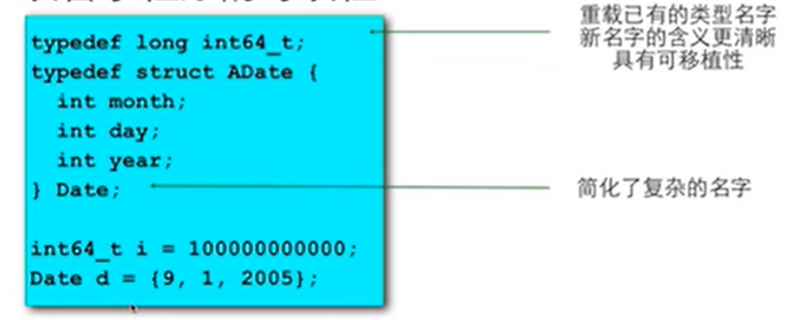### 13 联合或者联合体Union

• 结构体和共用体的区别在于：结构体的各个成员会占用不同的内存，互相之间没有影响；而共用体的所有成员占用同一段内存，修改一个成员会影响其余所有成员。
• 共用体占用的内存等于最长的成员占用的内存
• 文件操作，把整数等以二进制形式写入文件等可用
union var{
char c;
int i;
};

int main(){
union var data;
data.c = 0x04;//因为是char类型，数字不要太大，算算ascii的范围~
data.c = 0x03;//写成16进制为了方便直接打印内存中的值对比
data.c = 0x02;
data.c = 0x11;
//数组中下标低的，地址也低，按地址从低到高，内存内容依次为：04,03,02,11。总共四字节！
//而把四个字节作为一个整体（不分类型，直接打印十六进制），应该从内存高地址到低地址看，0x11020304，低位04放在低地址上。即为小端序
printf("%x\n",data.i);
}
typedef union{
int i;
char ch[sizeof(int)];
}CHI;
int main()
{
CHI chi;
int i;
chi.i =0x1234;
for(i = 0;i<sizeof(int); i++)
{
printf("%02hhX",chi.ch[i]);
}
return 0;
} 

### 14 变量

#### 静态本地变量

• 本地变量定义时加上static修饰符就成为静态本地变量
• 函数离开时，静态本地变量会继续存在并保持其值
• 静态本地变量的初始化只会在第一次进入这个函数时做，以后进入函数时会保持上次离开的值
• 是特殊的全局变量，与全局变量位于相同的内存区域
• 静态本地变量具有全局的生存期，函数内的局部作用域

#### 全局变量

• 定义在函数外面的变量是全局变量
• 全局变量具有全局的生存期与作用域，他们与任何函数都无关，在任何函数内都可以使用他们
• 没有初始化的会得到默认0值，只能用编译时刻已知的值来初始化全局变量，初始化发生在main函数之前
• 如果函数内部存在与全局变量同名的变量，则全局变量会被隐藏
• 尽量不要用全局变量来在函数间传递数据

#### static

一、 static全局变量与普通的全局变量有什么区别 ?全局变量(外部变量)的说明之前再冠以static 就构成了静态的全局变量。

static全局变量只初使化一次，防止在其他文件单元中被引用;

static局部变量只被初始化一次，下一次依据上一次结果值；

static函数与普通函数作用域不同,仅在本文件。只在当前源文件中使用的函数应该说明为内部函数(static修饰的函数)，内部函数应该在当前源文件中说明和定义。对于可在当前源文件以外使用的函数，应该在一个头文件中说明，要使用这些函数的源文件要包含这个头文件.
static函数在内存中只有一份，普通函数在每个被调用中维持一份拷贝。

### 15 宏定义

1. 宏定义

• #开头的是编译预处理指令，他们不是C语言的成分但C离不开它们
• #define用来定义一个宏，#define 名字 值， 值可以是任何东西，也可以是语句或者表达式。没有结尾分号因为不是C语句
• C的编译器开始编译之前，编译预处理程序会把程序里的名字替换成值，是完全的文本替换。
• 如果一个宏的值超过一行，最后一行之前的行末要加\
• 没有值的宏可以用来做条件编译。
• 预定义的宏_LINE_表示行数，_FILE_文件名，_DATE_,_TIME_指时间。

2. 带参数的宏

• 一切都要括号，整个值要括号，参数出现的每个地方都要括号：#define RADTODEG(x) ((x)*57.29578)
• 可以带多个参数，#define MIN(a,b) ((a)>(b)?(b):(a))
• 也可以组合或嵌套使用其他宏

### 16 头文件

• 把函数原型放在一个头文件（.h)里，在需要这个函数的源代码文件中#include这个头文件，就能让编译器在编译的时候知道函数的原型。
• #include是一个编译预处理指令，会把文件的全部文本原封不动的插入到他所在的那个地方，所以也不一定要在c文件的最前面#include
• 在定义和使用这个函数的地方都应该#include这个头文件，一般做法就是任何c都有对应同名的h，把所有对外公开的函数原型和全局变量的声明都放进去；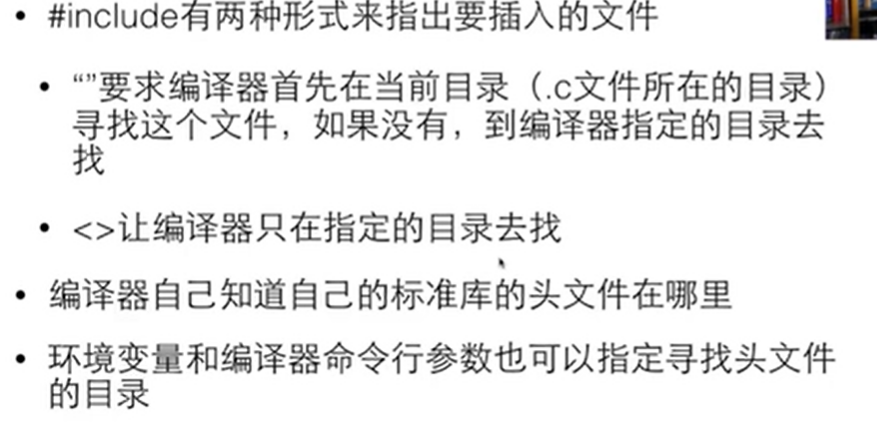• #include误区：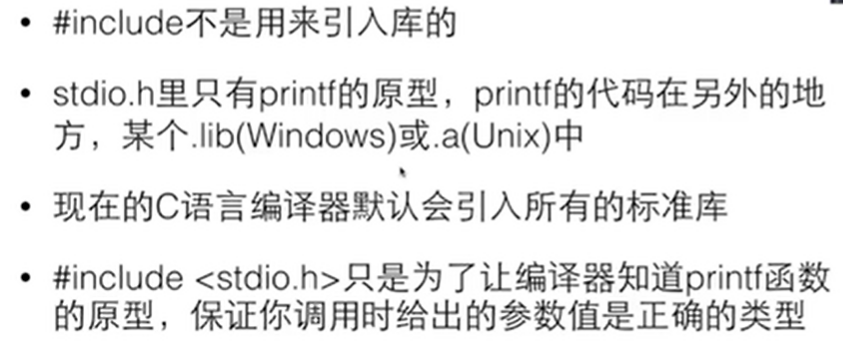• 函数面前加上static，函数就被定义成为静态函数，函数的定义和声明默认情况下是extern的，但静态函数只是在声明他的文件当中可见，不能被其他文件所用。
• 全局变量前加static，就使其成为只能在所在的编译单元中被使用的全局静态变量，全局静态变量在声明他的文件之外是不可见的。

### 17 声明

• int i;是变量的定义；extern int i;是声明
• 变量定义：用于为变量分配存储空间，还可为变量指定初始值。程序中，变量有且仅有一个定义。定义是产生代码的东西
• 变量声明：用于向程序表明变量的类型和名字。声明是不产生代码的东西。
• 声明：函数 ，变量声明结构声明，宏声明，枚举声明，类型声明，inline函数
• 只有声明可以放在头文件中
• 标准头文件结构中，运用条件编译和宏，保证这个头文件在一个编译单元中只会被#include一次。
#ifndef _UNION_CH_
#define _UNION_CH_
#include"un.h"
typedef union{
int i;
char ch[sizeof(int)];
}CHI;
#endif

### 18 标准输入输出

•  printf         %[flags][width][.prec][hiL]type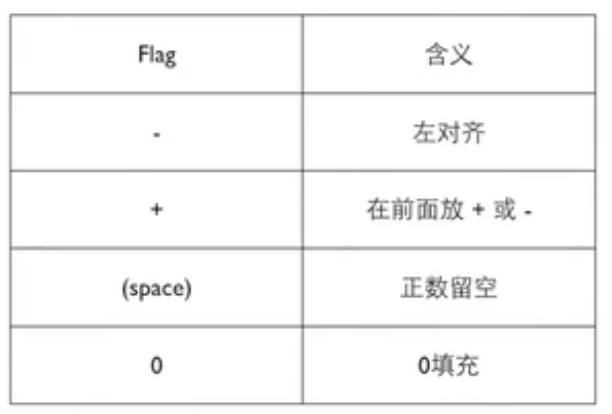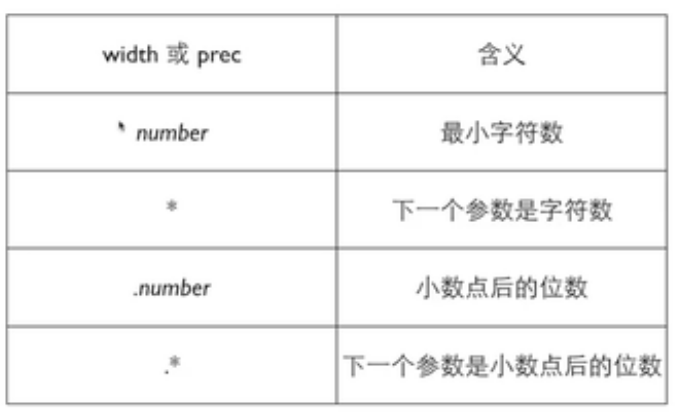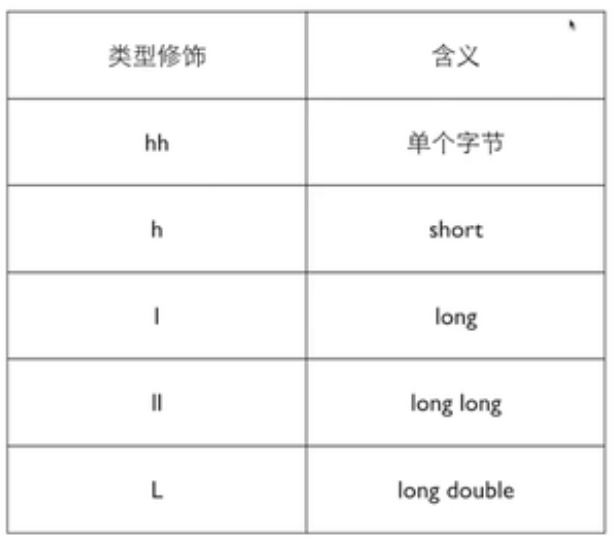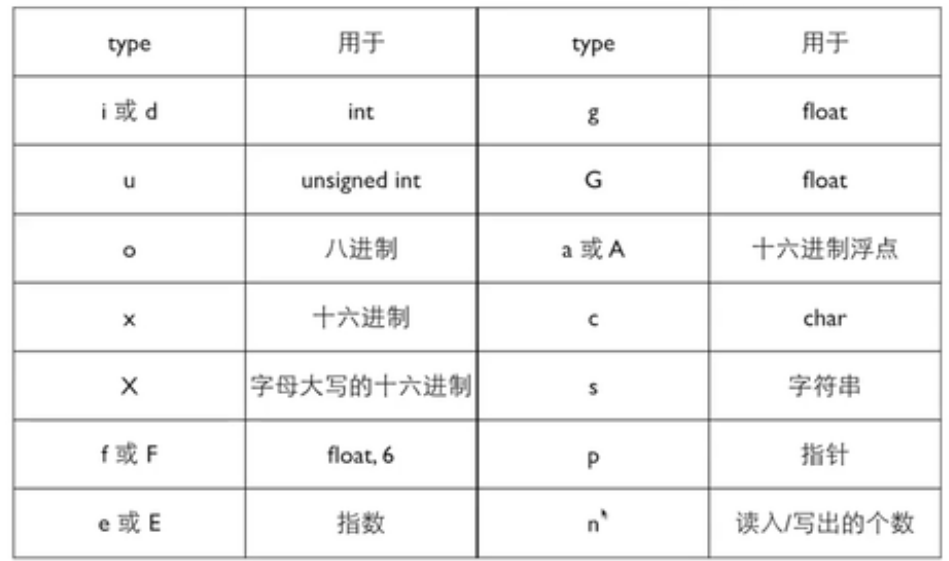•  scanf :   %[flag]type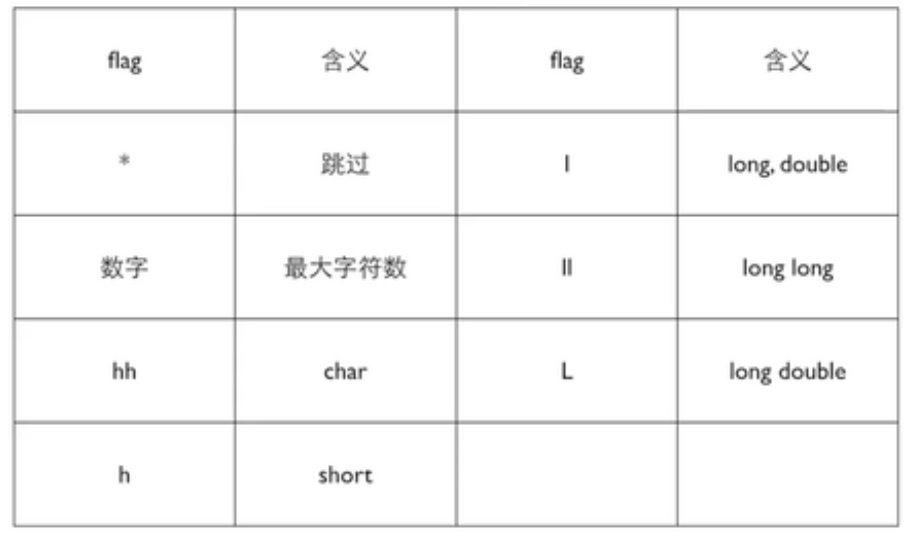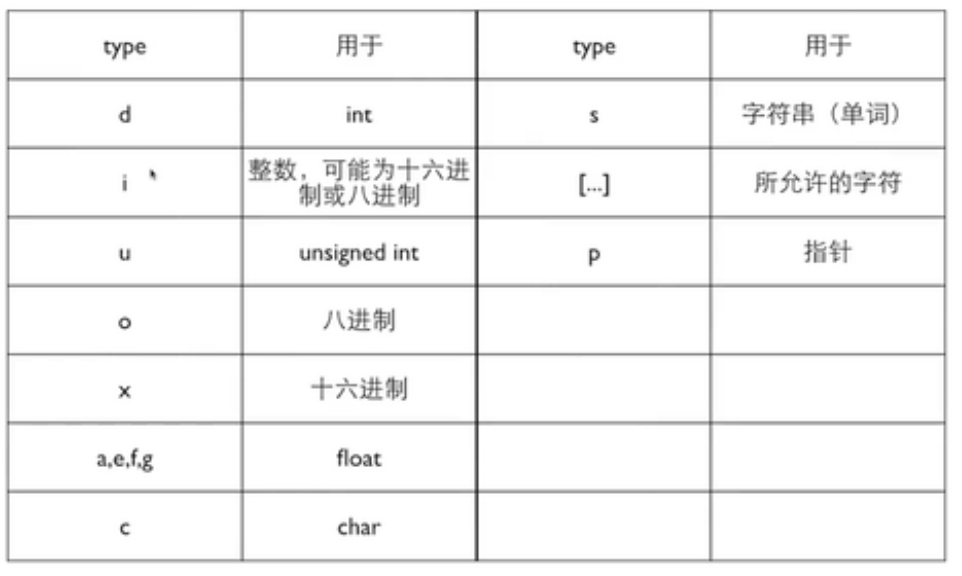•  printf返回输出的字符数，scanf返回读入的项目数，可以调用返回值看程序是否存在问题

### 19 输入输出

• 输入重定向即用文本文件代替键盘当作程序的输入。 ‘ < ‘ 符号是Unix、Linux和DOS的重定向运算符。该运算把文件和stdin流关联起来，将该文件的内容引导至程序。
• 输出重定向就是用文本文件代替屏幕当作程序的输出。’ > ’运算符是另一个重定向运算符。假设我们要将键盘输入的数据发送至一个名为test1.txt的文件。通过下面这条语句就可以完成：Reput.exe > test1.txt。
• FILE ，fopen等函数

### 20 文件读写

• 文本形式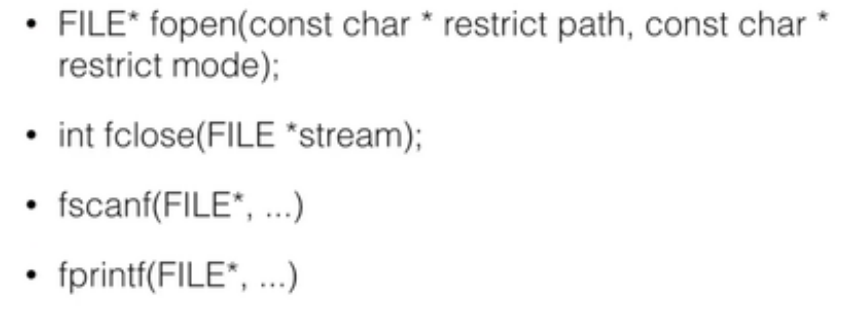•  二进制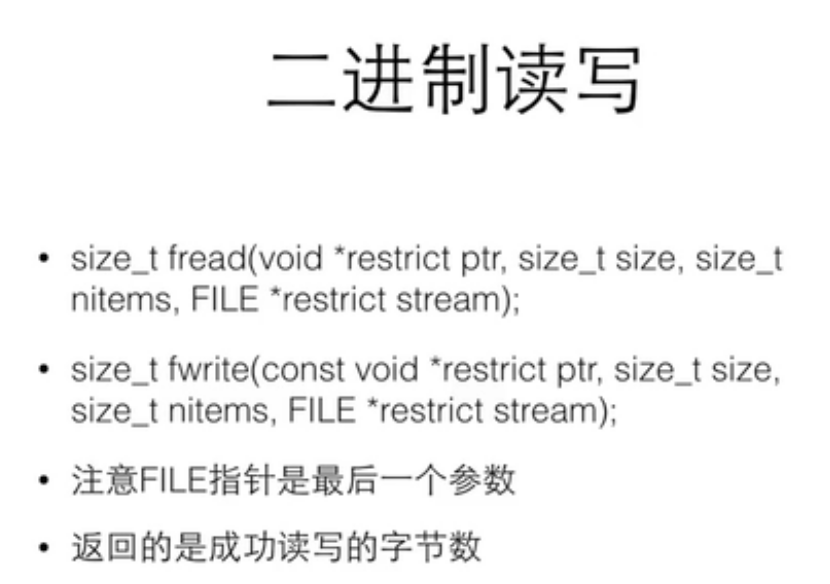• 比较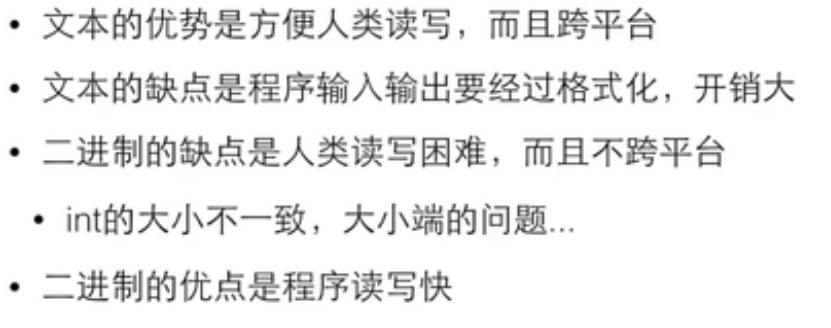• 可移植性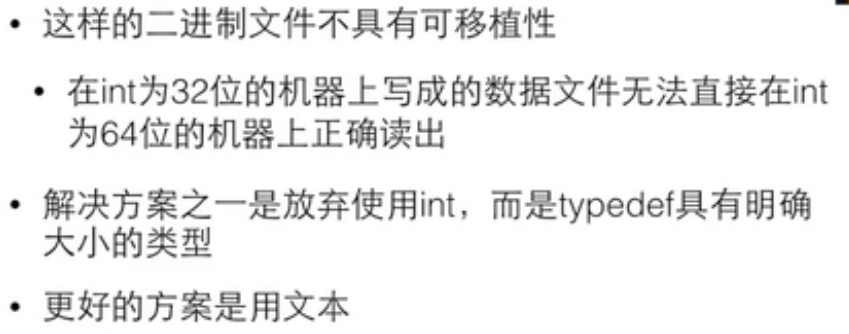### 21 按位计算

• 与&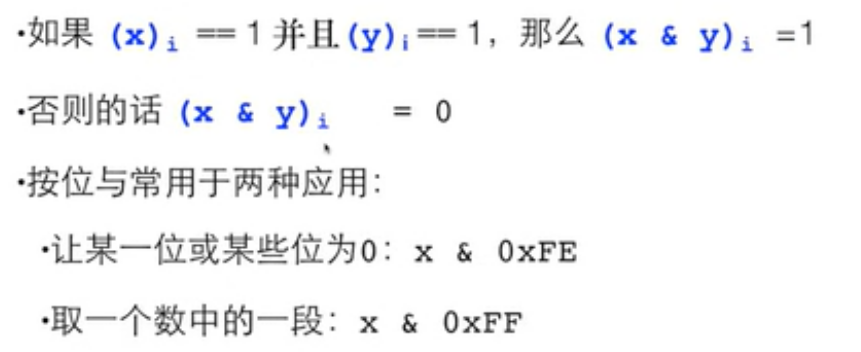• 或|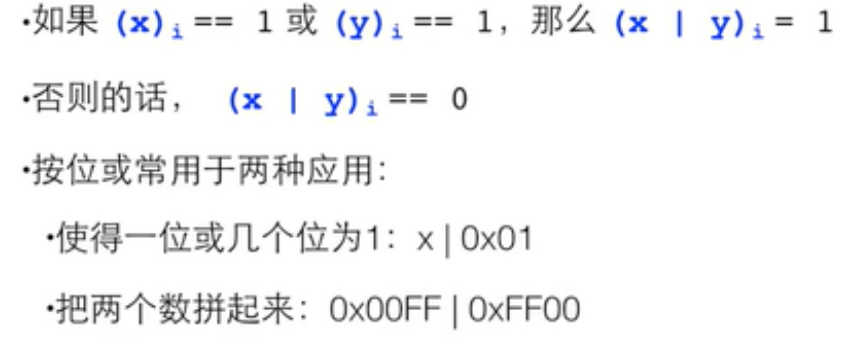• 按位取反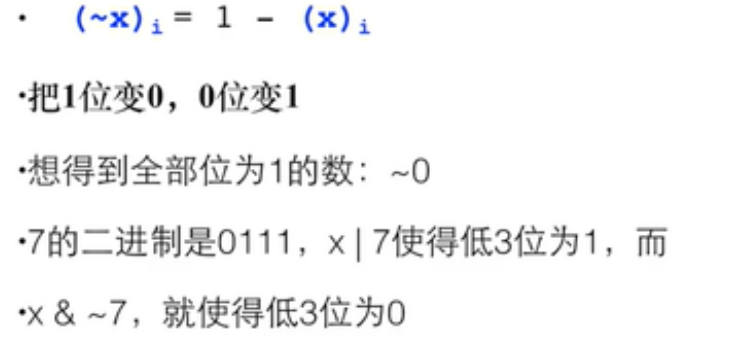•  按位异或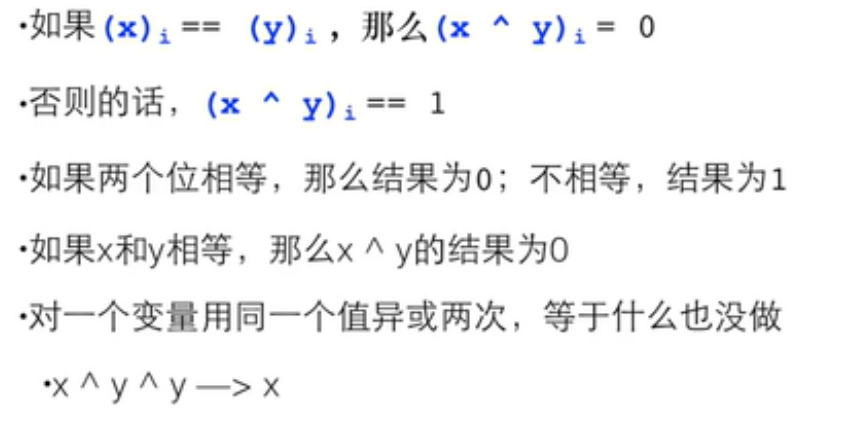### 22 位运算

• 左移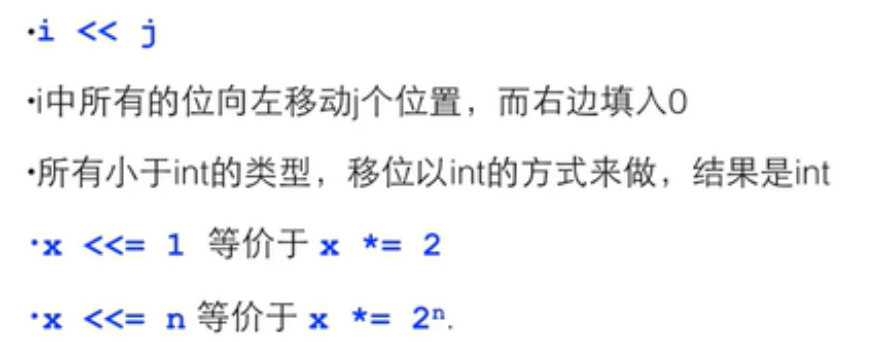•  右移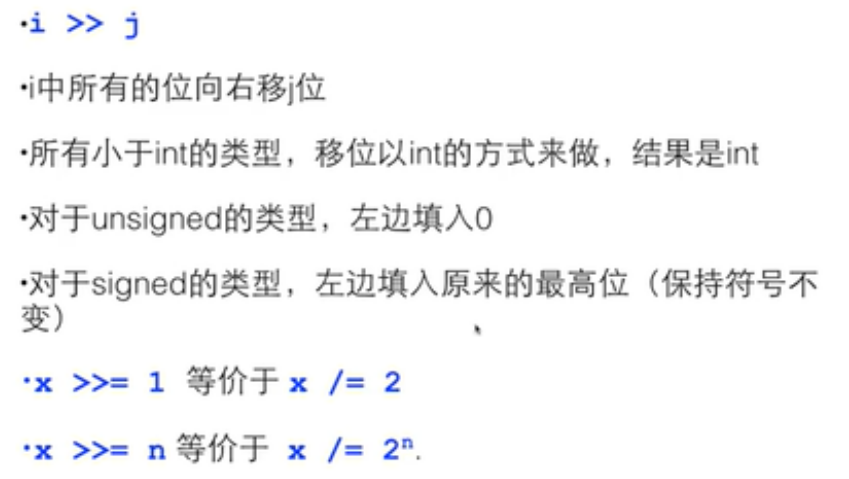• 位段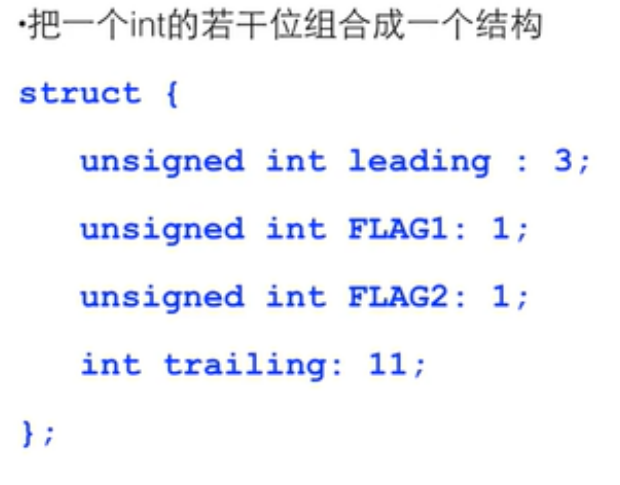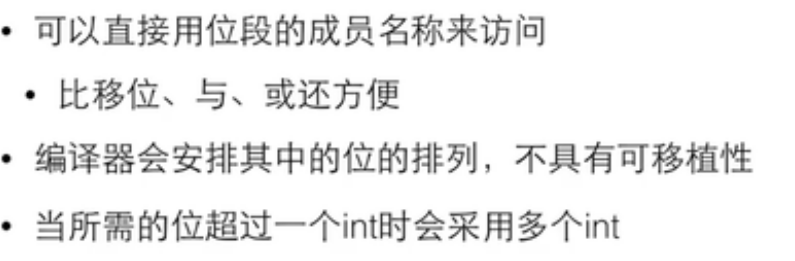### 23 可变数组

shuzu.h

#ifndef _SHUZU_
#define _SHUZU_
typedef struct{
int *array;
int size;
}Array;

Array array_create(int init_size);
void array_free(Array *a);
int array_size(const Array *a);
int* array_at(Array *a, int index);
void array_inflate(Array *a, int more_size);
void array_set(Array *a, int index, int value);
int array_get(const Array *a, int index);
#endif

shuzu.c

#include<stdio.h>
#include<stdlib.h>
#include<string.h>
#include "shuzu.h"

const int BLOCK_SIZE=20;

Array array_create(int init_size)
{
Array a;
a.size = init_size;
a.array = (int*)malloc(sizeof(int)*a.size);
return a;
}

void array_free(Array *a)
{
free(a->array);
a->array = NULL;
a->size = 0;
}

int array_size(const Array *a)
{
return a->size;
}

int* array_at(Array *a, int index)
{
if(index>=a->size)
{
array_inflate(a, (index/BLOCK_SIZE+1)*BLOCK_SIZE - a->size);
}
return &(a->array[index]);
}

int array_get(const Array *a, int index)
{
return a->array[index];
}

void array_set(Array *a, int index, int value)
{
a->array[index] = value;
}

void array_inflate(Array *a, int more_size)
{
int *p = (int*)malloc(sizeof(int)*(a->size + more_size));
//    for(int i = 0; i < a->size; i++)
//    {
//        p[i] = a->array[i];
//    }
memcpy(p, a->array, sizeof(int)*(a->size));
free(a->array);
a->array = p;
a->size += more_size;
}

int main()
{
Array a = array_create(5);
printf("%d\n",array_size(&a));
*array_at(&a, 0) = 10;
int cnt=0;
while(cnt<10)
{
scanf("%d", array_at(&a, cnt++));
}
for(int i = 0; i<cnt; i++)
{
printf("%d\n",array_get(&a, i));
}
array_free(&a);
return 0;
} 

###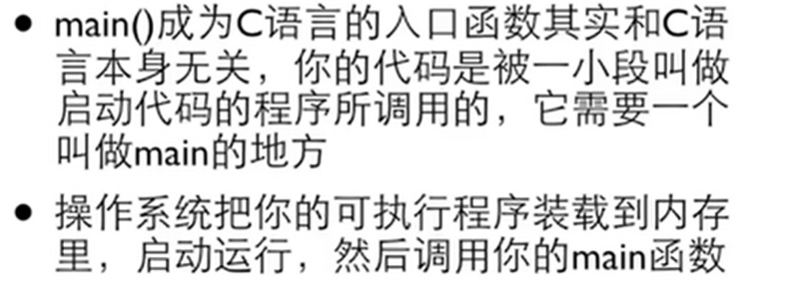#include<stdio.h>
#include<stdlib.h>
#include "shuzu.h"

typedef struct _node{
int value;
struct _node *next;
}Node;
typedef struct _list{
}List;
void add(List* pList, int number);
void print(List *pList);
int main()
{
int number;
List list;
printf("输入链表值，-1退出\n");
do{
scanf("%d", &number);
if(number !=-1){
}
}while(number!=-1);
print(&list);
printf("查找某个链表值\n");
scanf("%d",&number);
Node* p;
int isFound = 0;
if(p->value == number)
{
printf("找到了\n");
isFound=1;
break;
}
}
if(!isFound) printf("没找到\n");
printf("删除某个链表值\n");
Node* q;
for(q=NULL, p = list.head;p;q=p, p=p->next){
if(p->value == number)
{
//在->的左边的任何指针必须被检查
if(q)
{
q->next=p->next;
}
else
{
}
free(p);
break;
}
}
print(&list);
printf("释放链表值\n");
{
q = p->next;
free(p);
}
return 0;
}
void add(List* pList, int number)
{
Node *p=(Node*)malloc(sizeof(Node));
p->value = number;
p->next=NULL;
//find the last
Node* last = pList->head;
if(last){
while(last->next){
last = last->next;
}
last->next = p;
}
else{
}
}
void print(List *pList)
{
Node* p;
printf("打印链表值\n");
printf("%d\t",p->value);
}
printf("\n");
}

### 25 函数指针与回调函数

https://www.runoob.com/cprogramming/c-fun-pointer-callback.html

#include <stdio.h>
typedef int (*fun_ptr)(int,int);
int max(int x, int y)
{
return x > y ? x : y;
}

int main(void)
{
/* 函数指针 */
fun_ptr fun1;
fun1 = &max; // &可以省略
int a, b, c, d;
printf("请输入三个数字:");
scanf("%d %d %d", & a, & b, & c);
/* 与直接调用函数等价，d = max(max(a, b), c) */
d = fun1(fun1(a, b), c);
printf("最大的数字是: %d\n", d);
return 0;
}
• 回调函数：函数指针作为某个函数的参数

1. A "callback" is any function that is called by another function which takes the first function as a parameter。
2. 函数 F1 调用函数 F2 的时候，函数 F1 通过参数给 函数 F2 传递了另外一个函数 F3 的指针，在函数 F2 执行的过程中，函数F2 调用了函数 F3，这个动作就叫做回调（Callback），而先被当做指针传入、后面又被回调的函数 F3 就是回调函数。
3. 好处和作用，那就是解耦
#include<stdio.h>
#include<softwareLib.h> // 包含Library Function所在读得Software library库的头文件

int Callback() // Callback Function
{
// TODO
return 0;
}
int main() // Main program
{
// TODO
Library(Callback);
// TODO
return 0;
}

#include <stdlib.h>
#include <stdio.h>

void populate_array(int *array, size_t arraySize, int (*getNextValue)(void))
{
for (size_t i=0; i<arraySize; i++)
array[i] = getNextValue();
}

// 获取随机值,回调函数
int getNextRandomValue(void)
{
return rand();
}

int main(void)
{
int myarray;
/* getNextRandomValue 不能加括号，否则无法编译，因为加上括号之后相当于传入此参数时传入了 int , 而不是函数指针*/
populate_array(myarray, 10, getNextRandomValue);
for(int i = 0; i < 10; i++) {
printf("%d ", myarray[i]);
}
printf("\n");
return 0;
}
posted @ 2021-07-08 14:47  未闻花开  阅读(78)  评论(0编辑  收藏  举报